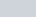ChemTalk

# Limiting Reactant and Limiting Reagent## Core Concepts – Limiting Reactant:

In this tutorial, you will learn what a limiting reagent is, how to find the limiting reactant, and see some example problems.

## What is a Limiting Reagent?

The limiting reactant is the reagent (compound or element) to be totally consumed in a chemical reaction. Limiting reactant is also what prevents a reaction from continuing because there is none left. The limiting reactant may also be referred to as limiting reagent or limiting agent. The reactant that is not used up is referred to as the excess reactant.

For example, consider if you are trying to put together a burger that has two pieces of bread, a piece of tomato, and a piece of meat. The reaction would be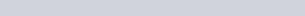You have 3 tomato pieces, 2 pieces of meat, and 6 pieces of bread.

When combined to make sandwiches, the first ingredient to run out is the meat. You only have enough meat to make two burgers but enough tomato and bread to make three burgers Therefore, the meat is the limiting reagent. The meat is the first ingredient to be used up. The bread and tomato have excess and therefore are called the excess reactant. The same idea is true for chemical reactions.

It is important to be able to find the limiting reagent because we can’t always add exactly the number of molecules we want to perfectly balance our equations. Often time, one reagent will be added in excess because it is cheaper and make sure the more expensive reagent is completely used up.

From the limiting reactant, the theoretical yield can also be calculated. The theoretical yield is the maximum amount of product that can be formed from the limiting reagent. However, the actual yield in a reaction is almost always less than the theoretical yield. Which is why the percent yield is calculated to compare the theoretical yield to the actual yield.

## How to Find the Limiting Reactant

There are several ways to find the limiting reagent. One key is to always start with a balanced chemical reaction. It is also essential to always compare mole amounts, not weight (ie grams). Only moles work for determining the limiting reagent because it tells us how many molecules there are.

#### Method 1 -Comparing Product Produced

For working through this method we will consider the reaction between nitrogen and hydrogen to make ammonia.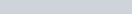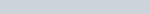2. Convert any amount given to moles of each reagent using stoichiometry.3. For each reagent, calculate how many moles of product would be produced.4. The reagent that produces the LEAST amount of product is your limiting reagent.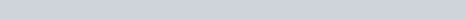Therefore H2 is the limiting reagent

#### Method 2-Comparing Reagent Available2. Convert any amount given (for example in grams) to moles of each reagent.3. Using the mole ratio, calculate the needed amount of product B to consume all A.4. If the calculated amount of B is higher than the actual amount of B that means B is the limiting reactant. If the calculated amount is less than the actual amount than B is NOT the limiting reagent.

The calculated amount of B: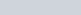The actual amount of B: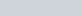Because the calculated B is greater than the actual B, we know H2 IS the limiting reagent in producing ammonia in this problem.

Notice that every method starts with balancing the chemical equation and then converting all amounts to moles. Then compare the mole ratios of either the product formed or the required amount of reagent.

## Example Problem of How to Find the Limiting Reagent

Problem: You have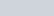and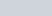to make water. Which is the limiting reactant in producing water?

Solution:

Start by balancing the chemical reaction.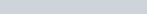Convert the amounts given in grams to moles.Calculate the amount of product produced by each reagentCompare the amount of product produced to therefore see which comes in the smaller amount.

The above result shows that oxygen (O2)  generates more water (H2O) than hydrogen (H2). Therefore, hydrogen acts as the limiting reagent.

## Limiting Reactant Practice Problems

Problem 1

You mix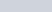of aqueous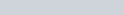with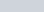of aqueousin excess acid. Which is the limiting reactant?

H2O2 and KMnO4 react according to the following equation: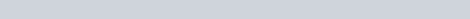In excess acid,

Problem 2

You mix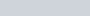into a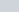aqueous solution of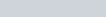. Assuming a 100% yield, how much AgCl would you expect to produce, in grams? (AgCl has a molecular weight of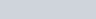).

CaCl2 and AgNO3 react according to the following equation: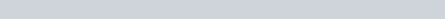## Limiting Reactant Practice Problem Solutions

1: H2O2

2: